Courses

# Types of Mirrors, Mirror Formula and Magnification Class 12 Notes | EduRev

## Class 12 : Types of Mirrors, Mirror Formula and Magnification Class 12 Notes | EduRev

The document Types of Mirrors, Mirror Formula and Magnification Class 12 Notes | EduRev is a part of the Class 12 Course Physics Class 12.
All you need of Class 12 at this link: Class 12

Mirror:

A smooth and highly polished reflecting surface is called a mirror.

(i) Plane Mirror: A highly polished plane surface is called a plane mirror.

Different properties of image formed by plane mirror:

Size of image = Size of object

Magnification = Unity

Distance of image = Distance of object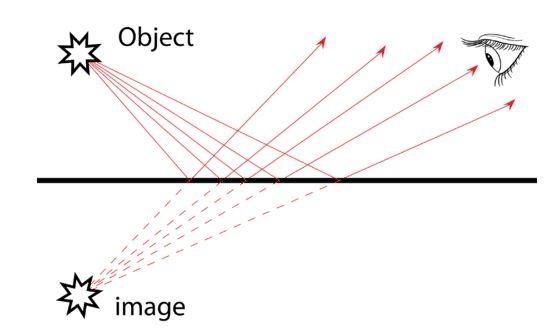Fig: Image formed by a plane mirror

A plane mirror may form a virtual as well as real image.

A man may see his full image in a mirror of half height of man.

When two plane mirrors are held at an angle θ, the number of images of an object placed between them is given as below:

(a) n = [(360° / θ) – 1 ], where 360° / θ is an integer.

(b) n = integral part of 360° / θ, when 360° is not an integer.

[A plane mirror may form a real image, when the pencil of light incident on the mirror is convergent. Children, during their play form an image of sun as wall by a strip of plane mirror.]

Kaleidoscope and periscope employ the principle of image formation by plane mirror.

If keeping an object fixed a plane mirror is rotated in its plane by an angle θ, then the reflected ray rotates in the same direction by an angle 2 θ.

Focal length as well as radius of curvature of a plane mirror is infinity. Power of a plane mirror is zero.

An image formed by a plane mirror is virtual, erect, laterally inverted, of same size as that of object and at the same distance as the object from the mirror.

(ii) Spherical Mirror: A highly polished curved surface whose reflecting surface is a cut part of a hollows at glass sphere is called a spherical mirror. Spherical mirrors are of two types:

(a) Concave Mirror: A spherical mirror whose bent in surface is reflecting surface, is called a concave mirror.

(b) Convex Mirror: A spherical mirror whose bulging out surface is reflecting surface, is called a convex mirror.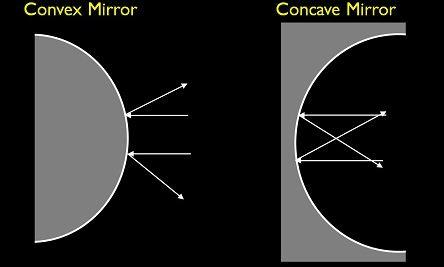Fig: Concave mirror vs convex mirrorSome Terms Related to Spherical Mirrors are Given Below:

(i) Centre of Curvature: It is the centre of the sphere of which the mirror or lens is a part.

(ii) Radius of Curvature (R): The radius of the hollow sphere of which the mirror is a part, is called radius of curvature.

(iii) Pole: The central point of the spherical mirror is called its pole (P).

(iv) Focus: When a parallel beam of light rays is incident on a spherical mirror, then after reflection it meets or appears to meet at a point on principal axis, which is called focus of the spherical mirror.

(v) Focal Length: The distance between the pole and focus is called focal length (f).

Relation between focal length and radius of curvature is given by

f=R/2

The power of a mirror is given as P = 1/f (metre)

(vi) Mirror formula: 1/f  = 1/v + 1/u

where, f = focal length of the mirror, u = distance of the object and v = distance of the image.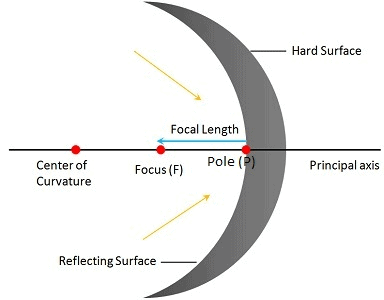Fig: Concave mirror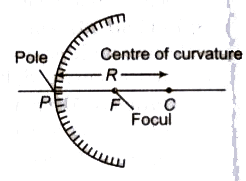Fig: Convex mirror

Newton’s formula for a concave mirror: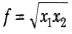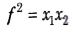where x1 and x2 are the distances of object and image from the focus.

Linear Magnification:

The ratio of height of image (1) formed by a mirror to the height of the object (O) is called linear magnification (m).

Lin ear magnification (m) = I/O = -v/u

Areal and Axial Magnification:

The ratio of area of image to the area of object is called areal magnification.

Areal magnification =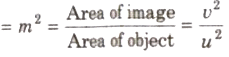When a small sized object is placed linearly along the principle axis, then its longitudinal or axial magnification is given by

Axial magnification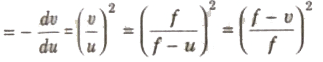Sign Convention for Spherical Mirrors:

1. All distances are measured from the pole of the mirror.
2. Distances measured in the direction of incident light rays are taken as positive.
3. Distances measured in opposite direction to the incident light rays are taken as negative.
4. Distances measured above the principal axis are positive.
5. Distances measured below the principal axis are negative.

Lateral lnversion:

In the image formed by a plane mirror the right side of the object appears as left side and vise-versa. This phenomena is called lateral inversion.

When object is placed between pole and focus of a concave mirror, then its virtual, erect and magnified image is formed.

A convex mirror forms a virtual, erect and diminished image for all conditions of object.

The focal length of concave mirror is taken negative and for a convex mirror taken as positive.

Offer running on EduRev: Apply code STAYHOME200 to get INR 200 off on our premium plan EduRev Infinity!

,

,

,

,

,

,

,

,

,

,

,

,

,

,

,

,

,

,

,

,

,

,

,

,

;